# Options Greeks: A Simple Guide to Learn

## Options Greeks are a set of mathematical functions that describe the price movements of options. They can use them to hedge positions and speculate on changes in the market. This blog post will provide an overview of how each of the option greeks works and how it influences an option's price movement.## 1. What are the greeks?

The price movements of options are directly related to five mathematical functions that fluctuate daily. A proper understanding of these can be advantageous, allowing traders to play the greeks in their favor instead of playing against them. There are five primary greeks: delta, gamma, theta, vega, and rho. Each Greek measures a different aspect of an option's price movement. Inside this blog post, we will go in-depth on each greek and its effect on the options you might be buying or shorting. This article will also overview specific option strategies and scenarios where you can use the greeks in your favor.

Each greek has a range from +1 to -1. A positive greek will increase the value of the option contract price when the underlying factor rises. For options traders to know how much they affect the options price, we will need to multiply each one by 100 because each option contract can buy or sell 100 shares depending on whether you are holding a call option or put option.

## 1. How the greeks options affect the price of the underlying

The price of an option is calculated through all five greeks, delta, gamma, vega, and rho. An option's value can be split into two different categories that account for all of the current value.

Options value = Extrinsic value + Intrinsic value

## Intrinsic value explained

The intrinsic value is the price of an option at the time of expiration which can help traders know how much weight the option has to lose. Intrinsic value can only exist if there is a difference between your strike and market price on the expiration date. This option would be considered expiring in the money. Therefore, it is the only value left if you were to exercise your option contract at any given time before or on the time an option expires.

Traders need to know what type of options they are buying because it can affect how much their position will gain or help minimize significant risk based on changes in the market price and how much it will cost them. If you are buying options, the only greek that matters is delta because its value directly affects your position's overall price changes and, therefore, performance.

On the other hand, if you were to sell an option against a long stock position, then gamma would be vital since it can determine how fast or slow your stock position will move.

## Extrinsic value explained

One of the main factors affecting an option's price is its extrinsic value. Extrinsic value can be thought of as the time value of money. The option price's extrinsic value decreases quickly as the expiration date nears and is extremely large the further away we are from expiration. An option is mainly a vehicle to gain leverage on a particular asset but the downside is paying a premium to hold this leverage vehicle mainly due to time decay. Time is the most significant value on any option and decreases the value very quickly as expiration nears.

## Intrinsic value example

This Facebook option is a price of \$4.95 currently has seven days till expiration, giving it a certain amount of extrinsic and intrinsic value. We will break those two values up so you can know the true risk of holding an option like this.

First, to calculate intrinsic value, we need to know the underlying's price, which is \$335.62. Because this number is higher than the strike price, we know these options are considered deep in the money.

To calculate the intrinsic value, you must subtract the same strike price by the underlying stock price, which is \$335.62-\$335 = \$0.62. This is the actual value of the option, and everything else over this is considered extrinsic value, which is the premium you are paying to hold this option. So to calculate the extrinsic value, we subtract \$0.62 by the option price, which is \$4.95, which means the value is around \$4.95-\$0.62 = \$4.33.

This number is a mix of time decay, volatility of the underlying, and more. For example, if you were to hold this option for the next seven days and the underlying price didn't move at all, you would be losing all \$433 and only have \$62 at the time of expiration, which comes down to a \$86 loss per day if we exclude the weekend.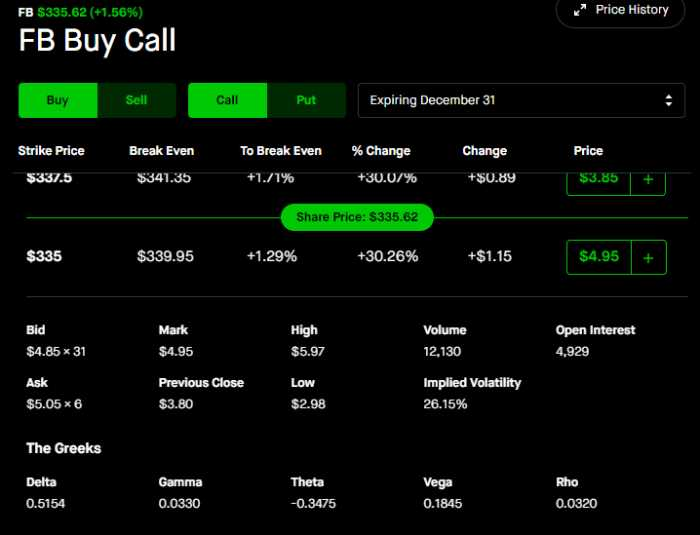This example same option with an extra week till expiration, which has a value of \$755, and an additional year till expiration is \$4,863. All three of these options have the same intrinsic value of \$62 dollars, but the further your expiration date, the more extrinsic value you add to the option.

Traders or investors will buy these options to speculate on price movements, but the percentage change amount they can make it smaller and smaller the further the expiration date because the price increases while the amount of leverage doesn't. Option sellers see the opportunity and look to put risk factors like time decay in their favor and hedge portfolios in certain situations.

##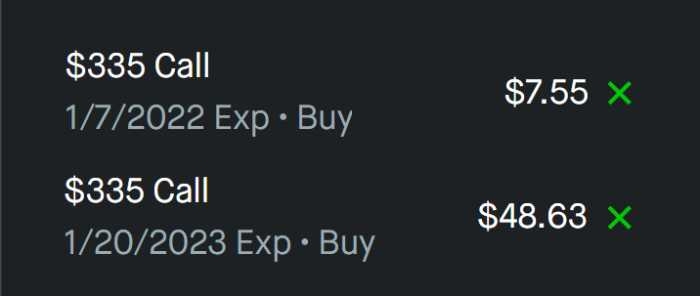Example of buying an option to take advantage of the greeks

In the example below, you can see the greeks column on Robinhood at the bottom for the Facebook 12/31 call option with a strike price of \$335 and \$495 (because we have to multiply it by 100). The positive greeks will be delta, gamma, vega, and rho. The factors related to these greeks will increase the options price if those factors increase.

For example, Delta is related to the price of the underlying stock. So when the price of the underlying goes up \$1 the price of the option should increase as well. The amount it increases will be 0.5154 to the option price, which would be \$4.95+\$0.5154 =\$5.4654 multiplied by 100, = \$546.

This means your option increased around 10% in value of \$51 because of the \$1 move in the price of the underlying. We will go more in-depth on these greeks later, but it is essential to note + greeks add to the call option value, and negative greeks decrease the value of the option.

The only negative greek in the example here is theta related to value lost per day, which is around \$0.34 x 100 = \$34. So every single day, this option can expect to lose that much money.

## Example of shorting an option to take advantage of the greeks

When shorting options, you can take advantage of the negative greeks and the factors where certain greeks decrease the option price. This risk management means going short on anything means you are borrowing the option with hopes of the value decreasing. To make money from short, the option's value needs to drop.

So while all the greeks are unchanged, staying the same, positive and negative, compared to the previous example, as the option is shorter, you can profit from the time value now. As well as when the factors affecting gamma, vega, delta, and rho decrease.

In this options trading investment strategy of how much delta changes, If delta increases the option price when the price of the underlying goes up, as the options seller, you would hope for the price of the underlying to drop in price since the delta also can decrease the value of the option by the same amount.

For example, when the price of the underlying goes down \$1 the price of the option decreases as well. The amount it reduces will be 0.5154 to the option price, which would be \$4.95-\$0.5154 =\$4.44 multiplied by 100, = \$444. This means your option decreased around 10% in value or \$51 because of the \$1 move in the price of the underlying.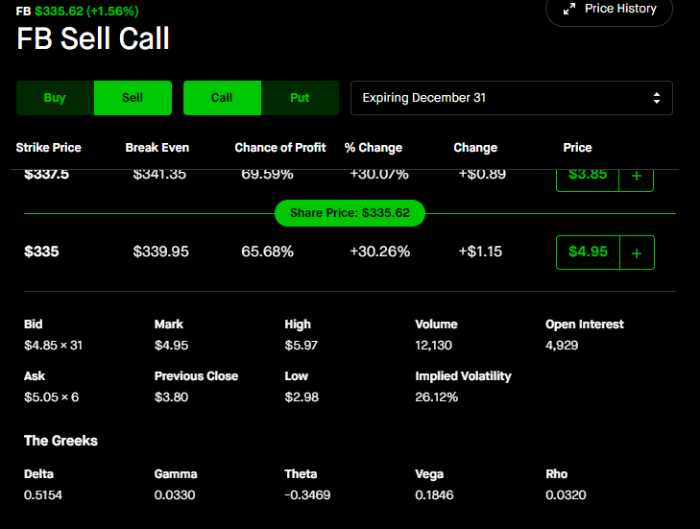## 2. How delta affects an option

Delta value is the rate of change in an option's price, given a \$1 move in the underlying security, and ranges from +1 to -1. Call options have a positive delta which means the cost of the option increases with the price of the stock. Put options have negative deltas, which means the option's price increases when the stock price moves downward.

## How delta changes with a strike price

The delta of an at the money (ATM) call option is around 0.50, in the money (ITM) call option is above +0.50-1.00, and lastly, out of the money (OTM) call options are between 0-0.5. Lower delta options can be seen as incredibly risky since they contain much extrinsic value and move very little per dollar in the underlying stock.

As you can see in the example below, at the money options offer the best leverage per dollar move, and the further you move up or down the option chain, the less of a percentage change increase you get compared to the value of the option.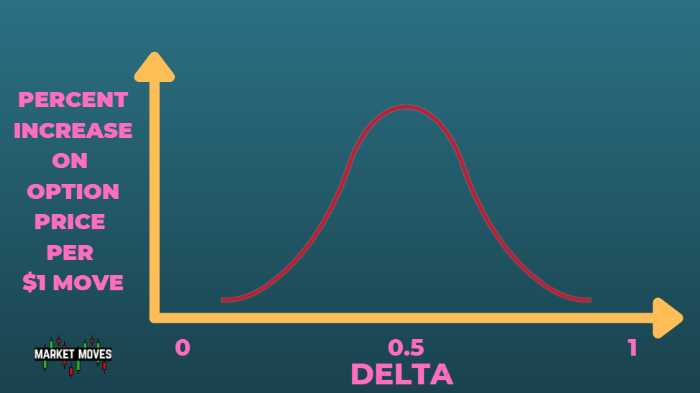An option's delta can also be expressed regarding the percentage chance of expiring in or out of the money. So an option with a delta of 0.50 would have a 50% chance of ending up in the money at expiration (it would increase \$0.50 if the underlying asset increased by \$100).

As discussed in the example above, the percentage affect delta has gotten smaller as the expiration nears.

## Delta to strategy

Some traders recognize the amount of leverage they can receive and try to take advantage of mispriced options that offer a higher intrinsic value than extrinsic value.

In the Facebook example above, the percentage of extrinsic value is around 85%. That is the amount of the option working against you through time value and volatility of the underlying drops. It is essential to note for risk measures, the higher this percentage, the harder it can be to profit from a trade.

Some traders will look towards low volatile stocks that will soon experience high volatility of the underlying. Currently, at this moment in the market, a very quiet dividend stock called AT&T has moved around 10%, which doesn't happen often.

If we look a the percentage of extrinsic value from at the money options, we can see the price of the stock is \$24.90, and the strike price is \$24.5. Subtracting these numbers, we get \$0.40, which is the option's intrinsic value. So the amount of extrinsic value is around 20%.

You are paying much less for the leverage when you are options trading. The option buyer pays around four times more for the same leverage for Facebook at the money options. Some traders will scan to see price changes and search for these options to give them a better chance to profit from a trade.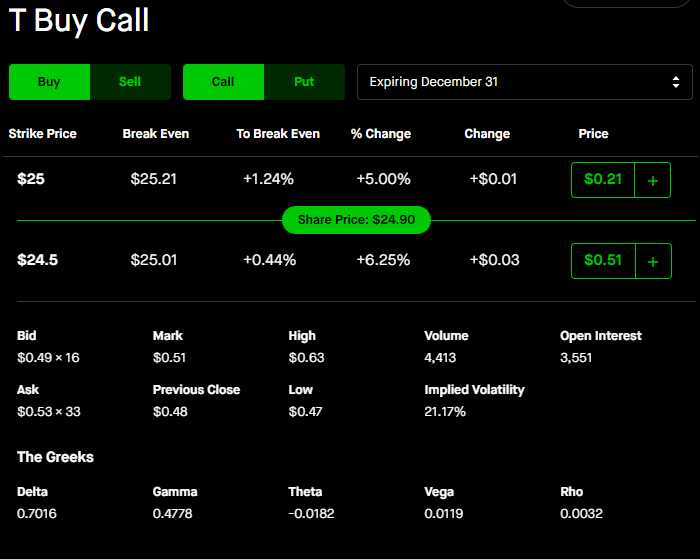## 3. Theta - time value

Theta measures how much option prices will change given the passing of one day. It is sometimes called time decay or time value because it works against you as days go by (or in favor if you are shorting options).

Imagine that you have a one-month option with a theta of with a theoretical value -0.05. This means that, on average, the option's price will decrease by \$5 each day. If we started with an option priced at 0.50 and it had a theta of -0.05, it would be worth 0.25, losing 50% of its value in one week. This is because most of the option's value is made up of extrinsic value.

Theta is also expressed in terms of percentage. So an option with a theta of 0.05 would lose (on average) or 10% of the option's value, which only increases closer to expiration (0.05/0.50 = 10%).

The amount of decaying time value has a positive relationship further and further. The options are out of the money and the reverse, where the time decay amount decreases significantly the further and further options are in the money.

An option that expires out of the money is considered gambling because they are entirely of extrinsic value, which again means they will have no value at the time of expiration. Which again means the options are going to zero. This is great for option sellers but extremely dangerous for option buyers. A choice that will expire in the money is ideal for options traders.

## Minimal decaying time value options

Options with a small amount of time decay as a percentage of the option value can offer a lot less risk to option traders. In the example above, AT&T offered a tremendous amount of intrinsic value at 80% of the option's value.

This is mainly due to how small the time decay is, at -0.0182 per day or around a 3% loss of the value of the option. If this is hard to stomach for holding options overnight, this amount will decrease as price changes go further and further in the money.In the example below. we can see the time decay remains similar at about -0.01 per day but as a percentage of the contract, it is almost not noticeable, being around 0.07% loss per day.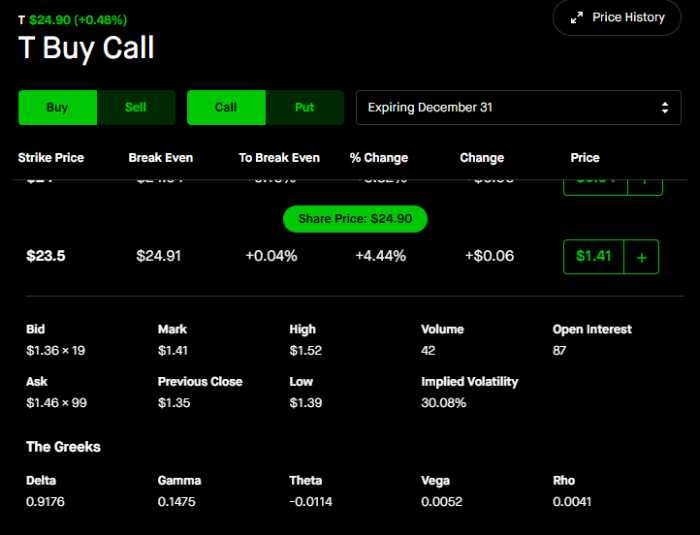## Use time decay in your favor

Time decay can be a nightmare or another trader's paradise if utilized properly. By selling options a trader can profit from the daily time decay of options. Certain options can pay a hefty premium per week if they go straight to zero and those options can be found on BreadAlerts.

BreadAlerts is a software that is an option seller's best friend, it scans the whole market every second to sort and filter for the highest possible premiums to sell. In the example below, if we sort the premium % column traders will be able to see the stocks containing the largest premiums to sell.

These premiums can range from 5 to even 15% some weeks. These can be considered options with a massive amount of extrinsic value compared to intrinsic value.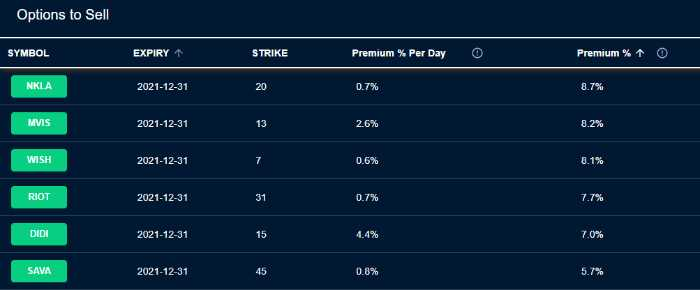In the example below, we can see BreadAlerts top option with the highest premium as a percentage of the capital needed is NKLA. If we head to the option chain on Robinhood we can see the 11.5 strike price put options is considered out of the money which means 100% of the value is extrinsic which means a large portion if not the whole amount could go to zero at the time of expiration. This option would be incredibly dangerous to sell because of this fact but the option seller has a huge opportunity if it in fact does expire worthless out of the money.

To sell a put option, the trader would need 100 shares per contract in case they needed to buy the shares for whatever reason. This amount would be \$1,150 and the amount the option seller could receive if the stock price stays the same for the next 7 days is \$94.

Which is a possible 8% return on their capital in one week. This example shows the dangers of being an option buyer especially holding options with a large amount of extrinsic value. In these cases, it is much safer to become an option seller.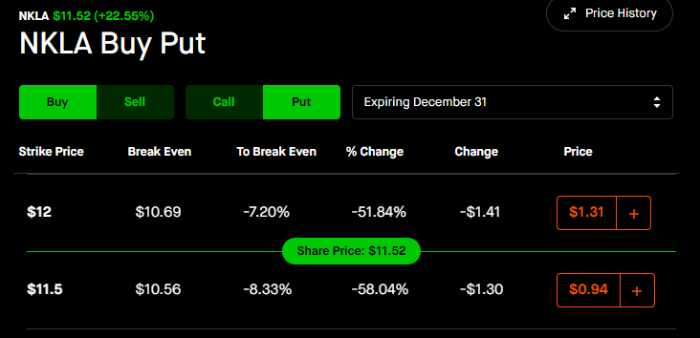## Time decay towards expiration

The decaying time value greatly increases as it gets closer to expiration when comparing it to the total percentage of the option value. As you can see in the example below, time decay is almost non-existent for options with more than 90-120 days till expiration. But the close and close we get towards expiration the faster options decay. This is the big worry holding options overnight with less than 10 days till expiration. Although this is an option seller's best friend because of how quickly value could be lost. Many option sellers will look to target options 7-30 days out because of this.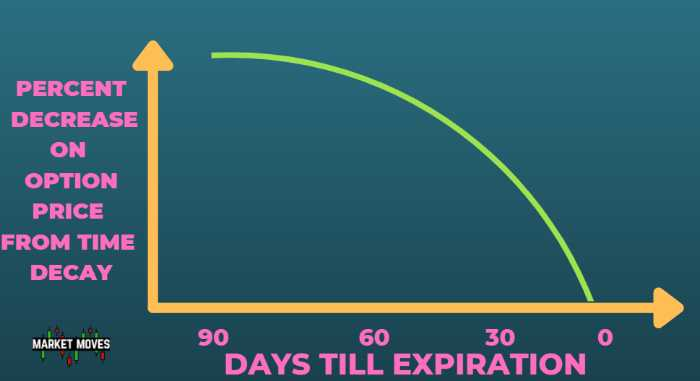## 4. Gamma - the rate of change in an option's price as a function of changes in underlying asset price and volatility

Gamma is the second-most important greek after delta. Gamma measures how much an option's Delta will change given a \$1 move in the underlying asset's price.

Since delta is the amount the option's price increases in value, a higher delta would mean more money per \$1 move. So an increase in delta is very beneficial to an options trader and a higher gamma will allow that.

For call options, if the price of the stock increases then gamma will be added to the delta. For put options, if the price of the stock decreases then gamma would be subtracted from the delta. Since delta is negative for put options, this would still mean a higher delta. This is good for an option holder because put options make money when the stock decreases in value.

## Gamma Example

In our recent Facebook example, if we were to understand the impact of gamma per dollar move we would look at the greeks on our options trading platform. We can see that the 335 strike price Facebook option has a gamma of 0.033 which means for a \$1 move at this specified price in the stock 0.033 would be added to the delta of 0.5154.

So the resulting delta would be 0.5484, which means the next dollar move you would make \$54.84 instead of \$51.54. This also means the delta would increase yet again for that second dollar move to possibly 0.5814. So a third dollar move would yield \$58.14, and so on.## Out of the money gamma exposure

Traders will utilize the gamma increases from out of the money options. Out of the money options can be the riskiest to hold but also have the greatest potential to increase massively in price given a big move in the underlying asset. This increase of gamma is the largest out of the money and does lead to those options moving more as a percentage of at the money options or in the money options.

In the example below, we can see NKLA ran 25% in one day from the underlying stock and the option percent gains were the largest out of the money. The further and further the strike price was in the money the smaller the percent one day change was. This is a large attraction to the out of the money options and largely due to gamma exposure.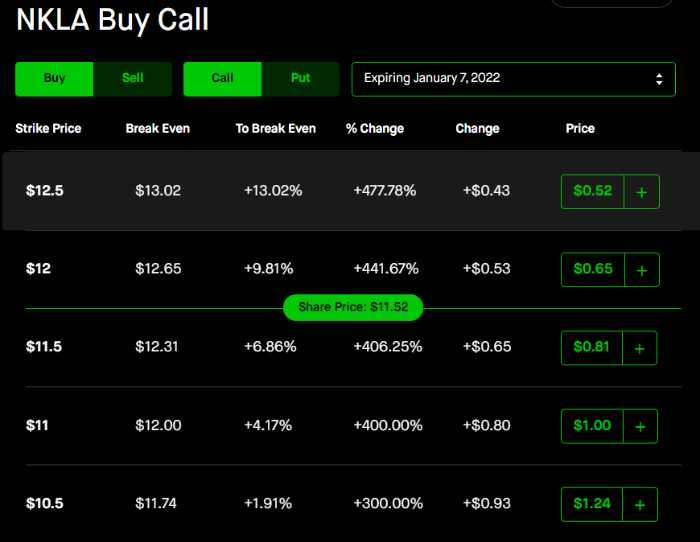## 5. Vega - sensitivity to changes in implied volatility

Vega measures an option's sensitivity to changes in implied volatility. If vega is a positive number, a move higher in volatility from the underlying asset increases the price of the option or vice versa for a negative vega value. We don't really see negative vega value but we know that is the opposite for option sellers. They would be losing money if the option price happened to increase from vega.

## Dangers of vega

Volatile options can be incredibly dangerous to hold if volatility changes suddenly. If we pull up the greeks for NKLA we can see vega is around 0.0084 and the implied volatility is around 116%.

This means that every 1 percentage move in implied volatility higher adds 0.0084 to the option and every 1 percentage drop in IV lowers the option's value by 0.0084. If NKLA happened to experience a 50% in IV, that would be detrimental for an option holder, as the value of the option could plummet 50 multiplied but 0.0084, or 0.42.

This is around 64% loss for option holders, even if the price happens not to move. This is the danger of trading highly volatile options and this is why option sellers will target these. These options have a large amount of extrinsic value and if they happen to not move for one or two days it could result is huge losses.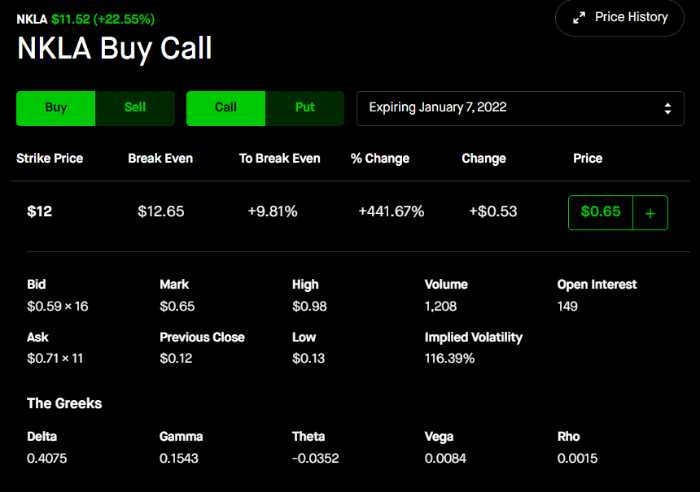## 6. Rho- sensitivity to the risk-free interest rates and dividends on the stock price

Rho measures an option's sensitivity to changes in the risk-free interest rate. It can be a positive number if the underlying asset is expected to move up and it will increase as the risk-free interest rate increases or vice versa for a negative Rho value.

An at the money call option has rho of -0.011 while ATM puts have 0.011 because they have positive and negative rho respectively.

An option's Rho can be expressed in terms of percentage (i.e., rho divided by 100) which is simply the Rho divided by Delta. So an option that has a rho of -0.00075 would have its delta change (on average) -0.000125 for every one percentage point change in the interest rates rise or fall.

## Rho has little impact

We are less worried about this option greeks option value as the movement of the fed funds rate has been very minimal for the past 20 years. From 2010 to 2015, the interest rates has been glued to zero which means there change in the options price for this period of time.

This greek has more an effect on options being held for 1-2 years, called leap options. During the 1980s to 1990s, the interest rates moved from 7 to 20% and then back down. This move in the interest rates would have had a much greater effect on options, especially ones being held for many months.## Risk free interest rate increase example

Despite the large swings in the interest rates, at the end of the day, Rho is still very small compared to the total option value. In the example below we can see CHPT has a Rho value of 0.07 which is around 1% value of the option price. The good news is since the interest rates are currently so low, any major increases to the fed fund interest rates would increase all long options being held.

If the interest rates were to increase by 5% the price of CHPT's 18 strike price option would increase by 0.35 to 6.35. This was calculated from 0.07*5 because Rho increases for every percentage the interest rate goes up. Overall that would increase the price of the options by more than 5% but the chance of that happening in one year would be extremely small. Because the fed is slowly increasing rates, we could see little movement in rho as this will have an effect on the underlying stock price.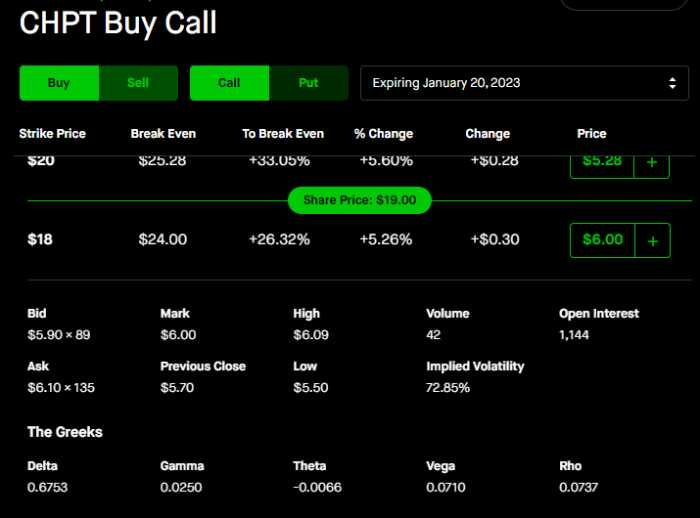## 7. Implied move calculations

We concluded that the options market is still very volatile and can be dangerous for traders who are not well versed. This volatility and measure move can be calculated daily from the price of an option. The implied move calculations are an easy way to see what the expected movement of the stock price is and can be very helpful when making trades. In the previous example, the implied move can be calculated from the price of the at the money options and multiply it by 2. The example above tells us that NKLA has an expected move of 0.65 * 2 = \$1.30. Which is around a 10% move in the stock alone. This can tell traders what the options market is pricing in and what could be expected. If this move fails to happen many of the options are going to lose a great amount of value.

## Conclusion - Wrapping it Up

The Greeks, or greeks as traders often refer to them, can be tricky and intimidating. But understanding how they affect the price of an option is key to knowing what type of options you should trade for your portfolio. Of course, we recommend consulting a professional before making any decisions about investing in this volatile market!